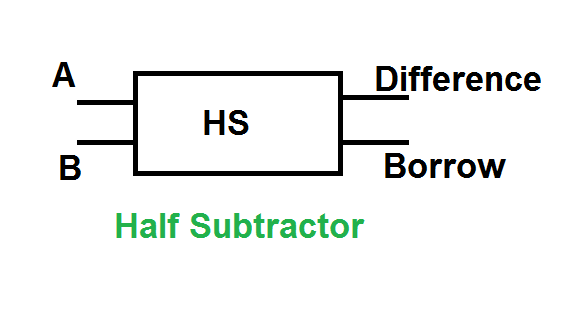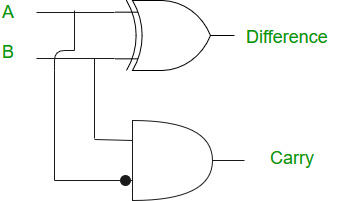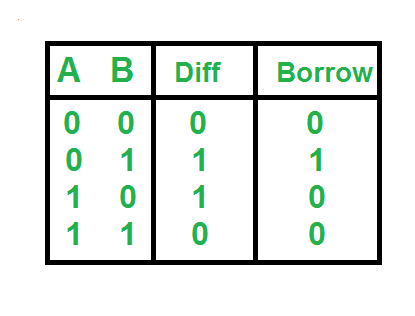# Python program to implement Half Subtractor

• Last Updated : 09 Jun, 2021

Prerequisite: Half Subtractor in Digital Logic

Given two inputs of Half Adder A, B. The task is to implement the Half Subtractor circuit and Print output i.e Difference and Borrow of two inputs.

The half subtractor is also a building block for subtracting two binary numbers. It has two inputs and two outputs. This circuit is used to subtract two single bit binary numbers A and B. The difference and borrow are the two output states of the half subtractor.

Examples:

Input: A=0; B=1

Output: Difference: 1

Borrow: 1

Explanation: According to logical expression Difference=A XOR B i.e 0 XOR 1 =1, Borrow=Ā AND B i.e 1 AND 1 =1

Input: A=1; B=1

Output: Difference: 0

Borrow: 1

### Logical Expression:

```Difference = A XOR B
Borrow = Ā AND B```

### Logical Diagram:### Truth Table:### Approach:

• We take two inputs A and B.
• XOR operation on A and B gives the value of the Difference.
• AND operation on Ā and B gives the value of Borrow.

## Python3

 `# Python program to implement Half subtractor`   `# Function to print Difference and Borrow` `def` `getResult(A, B):`   `    ``# Calculating value of Difference` `    ``Difference ``=` `A ^ B`   `    ``# Calculating value of Borrow` `    ``# calculating not of A` `    ``A ``=` `not``(A)` `    ``Borrow ``=` `A & B`   `    ``# printing the values` `    ``print``(``"Difference:"``, Difference)` `    ``print``(``"Borrow:"``, Borrow)`     `# Driver code` `# Inputs A ,B` `A ``=` `0` `B ``=` `1`   `# passing two inputs of halfadder ` `# as arguments to get result function` `getResult(A, B)`

Output:

```Difference: 1
Borrow: 1```
My Personal Notes arrow_drop_up
Recommended Articles
Page :